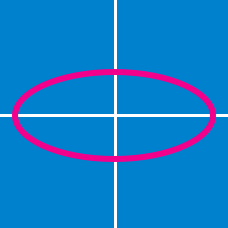Geometry

# Conics - Hyperbola

Consider a branch of the hyperbola $x^2-2y^2-2\sqrt{5}x-4\sqrt{2}y-3=0$ with vertex at a point $A.$ Also, let $B$ be one of the end points of its latus rectum. If $C$ is the focus of the hyperbola nearest to point $A,$ what is the area of triangle $ABC ?$

What are the coordinates of the foci of the rectangular hyperbola $x^2-y^2=7 ^2?$

What are the coordinates of the vertices of the hyperbola $4x^2-9y^2-40x+54y -17=0 ?$

Given points $F=(5,0)$ and $F'=(-5,0),$ what is the equation of the locus of points $P$ in a plane such that $\left\lvert \left\lvert \overline{PF} \right\rvert - \left\lvert \overline{PF'} \right\rvert \right\rvert= 8 ?$

Note: $\lvert \overline{AB} \rvert$ denotes the length of line segment $\overline{AB}.$

Suppose that $(7,12)$ and $(11,8)$ are the foci of a hyperbola passing through the origin. Then the eccentricity of the hyperbola is $\text{\_\_\_\_\_\_\_\_\_}.$

×